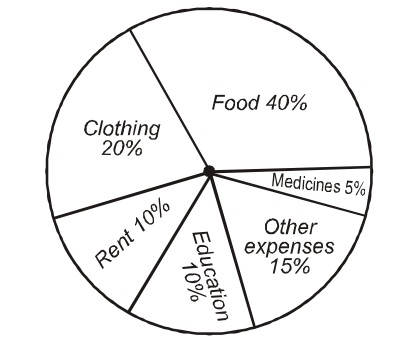Home » Data Interpretation » Pie chart » Question

#### Data Interpretation

Direction: The pie-chart given below shows the household expenditure of a family on different items. Study the chart carefully and answer the questions that follows.1. If total expenditure of this family is Rs. 4500, find the expenditure of the family on clothing?
1. Rs 800
2. Rs 900
3. Rs 840
4. Rs 950
##### Correct Option: B

As per graph,
Expenditure on clothing = 20 %
Expenditure on clothing = Total expenditure x 20 %
Expenditure on clothing = 4500 x 20/100 = Rs. 900
Expenditure on clothing = 45 x 20 = Rs. 900